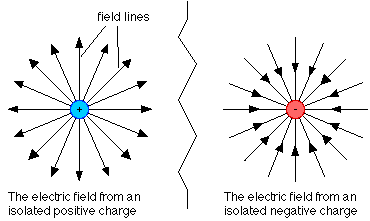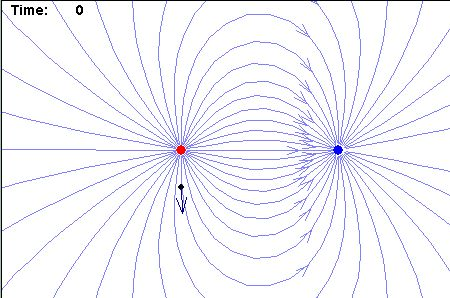# Introductory physics: the concept of field

In a first course in physics, it is usually in electrostatics that one first encounters the concept of a field.

Everybody knows that like charges repel, while unlike charges attract. The quantitative version of this rule is Colomb’s law, which is the observation that the force between two electric charges A and B is given by F  =  k.q(A).q(B)/r2 where q represents electric charge and r is the separation of the charges (k is is a constant determined by the medium in which the charges are situated). Note that if the charges are like, the force comes out positive, so a repulsive force is positive in sign, which is what you might expect since work must be done to bring the charges together. On the other hand, if the charges are unlike, the force comes out negative (which also makes sense as the charges want to be together anyway).However, the concept of force isn’t all that useful if one wants to know the effect of a given electric charge (A) on the world. It is clear from Coulomb’s law above that the force experienced by any charge B due to A will also depend on the magnitude of B i.e. each charge you bring up to A will experience a different force! Physicists get around this problem by defining the field due to charge A as the force a test charge brought up to A will experience divided by the magnitude of that test charge. Hence, while  every charge brought up to A will experience a different force, they will all experience the same field (F  =  k.q(A)/r2) – clever huh?

We can even draw pictures of the field due to A, simply by drawing lines representing the direction in which an electric charge will move. Unfortunately, the convention is that we draw the direction a positive charge will move (unfortunate because we now know that it is the negatively charged electron that’s doing the moving: the convention is historical)Electric field between two unlike charges (red is +ve)

The concept of a field is not limited to electricity; it is used throughout physics. For example, you and I experience slightly different forces due to the earth’s gravity. This is because the gravitational force depends on the product of both the earth’s mass M and personal mass m (F = GMm/r2 where G is a constant). However we all experience exactly the same field: dividing by personal mass, the gravitational field due to the earth is given by GM/r2.The earth’s gravitational field is directed towards its centre

Note

You may have noticed that the equation for gravitational force above looks very like that for the electric force, with charge replaced by mass (both forces decrease with the square of increasing distance). However, gravity is a much, much weaker force; the gravitation constant G is orders and orders of magnitude smaller than the electric constant k and you only notice a body’s gravitational field if it has the mass of a planet!

That said, it is now believed that there is a deep connection between electricity and gravity; indeed, particle physicists and cosmologists believe that all four of the fundamental forces of nature (gravity, electromagnetism and two nuclear forces) originally formed one superforce, which gradually split off into four separate forces as the uiverse expanded and cooled. We already have strong theoretical and experimental verification that two of the fundamental forces originally comprised one force, and it is one of the great ambitions of theoretical physics to describe all four forces in a single mathematical framework (unified field theory). Within that program, one of the great puzzles is why the gravitational force is so much weaker than all the others.

Filed under Introductory physics

### 6 responses to “Introductory physics: the concept of field”

1.Michael Schmitt

One of the early debates in the development of the theory of electricity and magnetism was whether these fields are *real* or just convenient mathematical devices. Eventually people were able to show that a pure electromagnetic field contains energy, and in some circumstances, linear and angular momentum. Experiments confirmed these deductions, thereby establishing the reality of such fields. When it comes to gravity, the bending of light by mass provides a similar, and even more profound, illustration.

2.durgadas datta

Deep interconnection of forces is very interesting when we see even model of atoms also have orbital 1/rxr ratio sequewnce. That means the exotic energy GEAND ETHER flowing over has a relation we have to find out from super spherical string theory developed by me . Even Avogadros law and Mendeleefs table can also be explained.

3.cormac

Micheal: exactly right, I should have included it!

4.irshad

thanks buddy

5.abilogun oluwatobi jesse

who are cosmologist,can i have some explaination of strong i interaction?

6.computer coupon program

You can certainly see your skills within the article you write.
The sector hopes for more passionate writers such as you
who are not afraid to say how they believe. At all